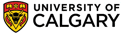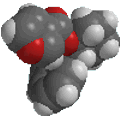Chapter 27: Amino Acids, Peptides, Proteins and Nucleic AcidsStructure and pKa of Amino Acids

• Even the simplest amino acids have both an acidic functional group, the carboxylic acid, and a basic functional group the amine.
• Compounds that can behave as both acids and bases are said to be amphoteric.
QUESTION Can you think of a very simple, common compound that is amphoteric ? ANSWER

The equations that define acidity and basicity are: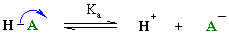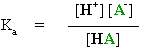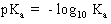Remember that the lower the pKa, the stronger the acid. ( review ?)

From these expressions it is possible to derive the important Henderson-Hasselbalch equation :

pKa = pH + log [HA] / [A-]

How does this equation help us ?

• It tells us that when the pH = pKa then log [HA] / [A-] = 0 therefore [HA] = [A-]   i.e. equal amounts of the two forms, the acid and the conjugate base.
• If we make the solution more acidic, i.e. lower the pH, so pH < pKa, then log [HA] / [A-] has to be > 0 so [HA] > [A-]. This makes sense as it tells us that a stronger acid will cause the formation of HA, the protonated form.
• If instead we make the solution more basic, ie raise the pH, so pH > pKa and log [HA] / [A-] has to be < 0 so [HA] < [A-]. This makes sense as it tells us that a stronger base will cause the formation of A- , the deprotonated form.
IMPLICATIONS :
• Typical simple carboxylic acids, RCO2H,  have a pKa of about 5, and typical simple ammonium ions RNH3+ have a pKa of about 9.
• Therefore, since the acid is the stronger acid (lower pKa) the amino acid will exist in the zwitterionic form where the acid has protonated the amine in neutral aqueous solution (or normal physiological pH).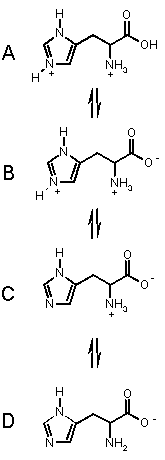These principles can be extended to poly acidic / basic systems (such as amino acids) by thinking of each pKa value in turn.
This information will let you decide which structure of an acid or base will dominate at a particular pH.

Let's look at an example.

To the left are the processes for the amino acid HISTIDINE which has an extra basic group.

It has three acidic groups of pKa's 1.82 (carboxylic acid), 6.04 (pyrrole NH) and 9.17 (ammonium NH).
Histidine can exist in the four forms shown, depending on the solution pH, from acidic pH (top) to basic pH. (bottom).

Starting from the top, we can imagine that as we add base, the most acidic proton is removed first (COOH), then the pyrrole NH then finally the amino NH. These takes us through each of the forms in turn.

At pH < 1.82, A is the dominant form.

In the range 1.82 < pH < 6.02 B is the dominant form.

In the range 6.02 < pH < 9.17 C is the dominant form, and

when pH > 9.17, D is the major form in solution.© Dr. Ian Hunt, Department of Chemistry